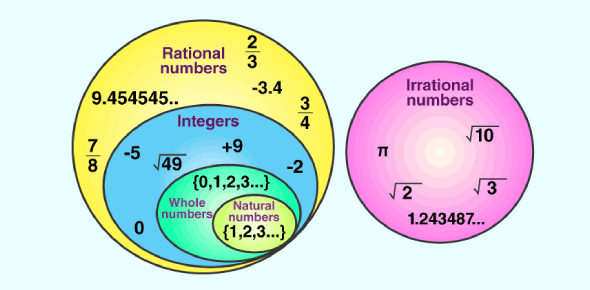# Rational Numbers Quiz: Math Exam!

9 Questions | Attempts: 1952Settings.

• 1.
0.45÷5=
• A.

0.9

• B.

9

• C.

90

• D.

0.09

• 2.
The equivalent rational number of -3/5.
• A.

9/15

• B.

-15/10

• C.

-6/15

• D.

-9/15

• 3.
The equivalent rational number for -12/30 with denominator 5.
• A.

2/5

• B.

-2/5

• C.

3/5

• D.

-3/5

• 4.
The increasing order of -3,-2,-5,0.
• A.

-5,-3,-2,0

• B.

0,-2,-3,-5

• C.

-2,-3,-5,0

• D.

-2,-3,-5,0

• 5.
The average of 4.5,2.7,3.3.
• A.

4.5

• B.

3.5

• C.

5.5

• D.

None

• 6.
Rational numbers are denoted by "R".
• A.

True

• B.

False

• 7.
Integers are denoted by "Z"
• A.

True

• B.

False

• 8.
5/6,1/6,7/6 are equivalent rational numbers.
• A.

True

• B.

False

• 9.
Every Integer is a Rational number.
• A.

True

• B.

False

## Related TopicsBack to top
×

Wait!
Here's an interesting quiz for you.